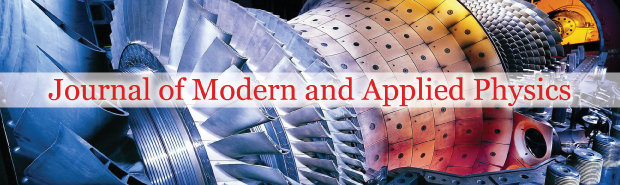All submissions of the EM system will be redirected to Online Manuscript Submission System. Authors are requested to submit articles directly to Online Manuscript Submission System of respective journal.### Gell-Mann matrices (strong force interaction) in geometric algebra Cl3,0

Author(s): Jesús Sánchez*

In this paper, we will find a way to apply the Gell-Mann transformations made by the λi matrices using Geometric Algebra Cl3,0. And without the need of adding the time as an ad-hoc dimension, but just considering that:The transformations are as follows. Considering the original ψ:The new ψ’ obtained when applying each of the Gell-Mann matrices λi is:Considering that Gell-Mann matrices do not consider at all the existence I ifand, it is possible that we should consider them zero form the beginning. Anyhow, above relations would correspond with the most general case.

We have also worked in the bra-ket product using geometric algebra. For the Euclidean case we have the equation (where the cross sign means reverse and the asterisk means conjugate, both mean the same in Cl3,0:Being ρ the probability density:Andthe fermionic current:But in this case:And:It has been also shown that the g-2 issue of the muon could be related to gravitational (non-Euclidean metric) issues without needing another natural force.

The difference of the values of g-2 of the muon are:And the effect of the non-Euclidean metric on the surface of Earth is:Full-Text | PDF

###### Journal of Modern and Applied Physics peer review process verified at publonsTop